## Mathematics teacher of a school took her 10th standard students to show Red fort. It was a part of their Educational trip. The teacher had interest in history as well. She narrated the facts of Red fort to students. Then the teacher said in this monument one can find combination of solid figures. There are 2 pillars which are cylindrical in shape. Also 2 domes at the corners which are hemispherical.7 smaller domes at the centre. Flag hoisting ceremony on Independence Day takes place near these domes.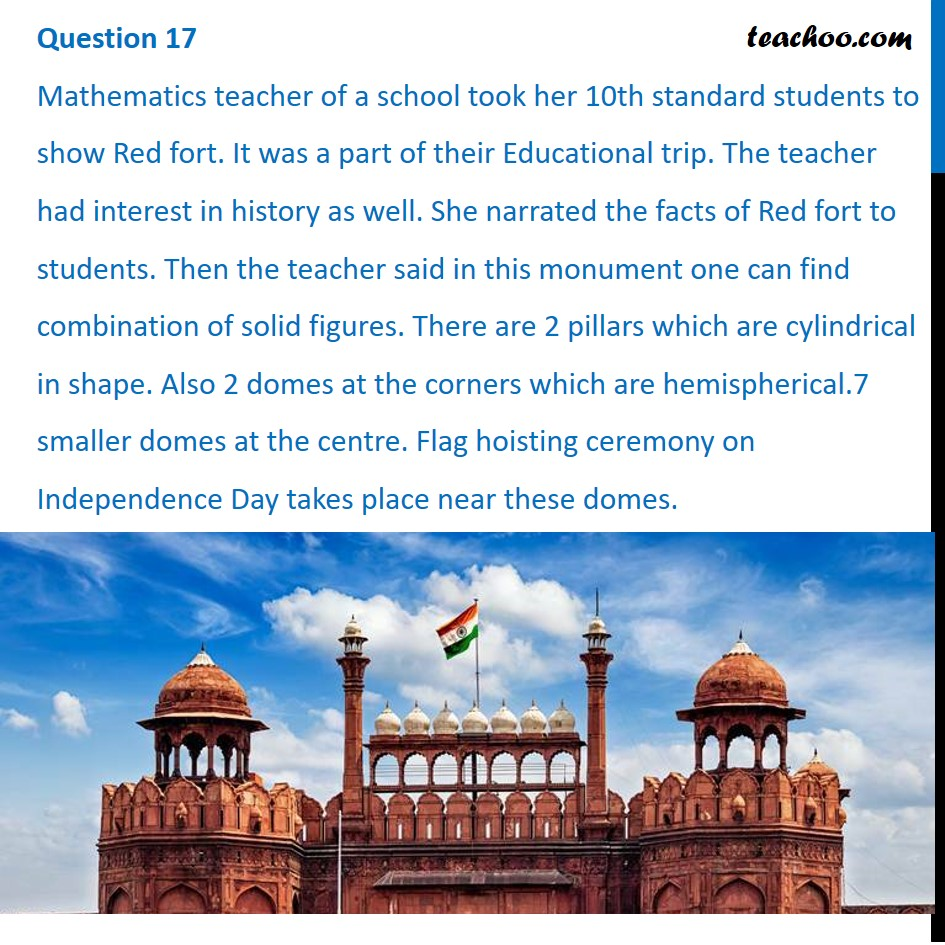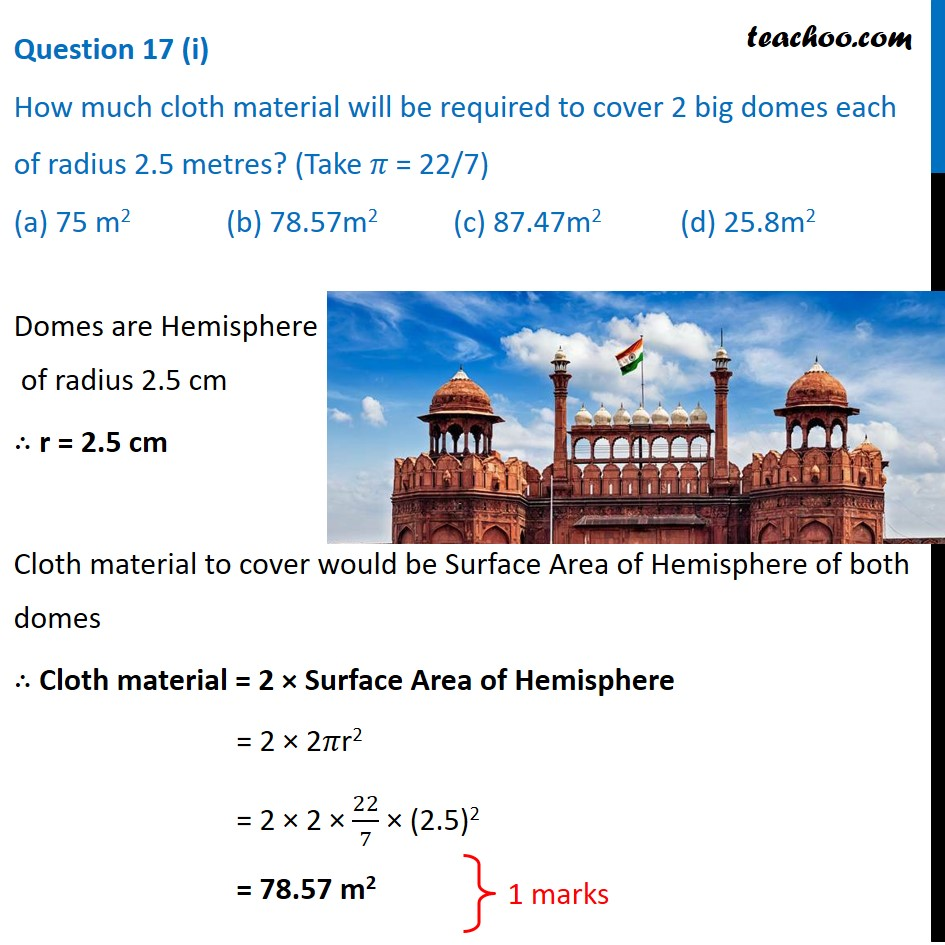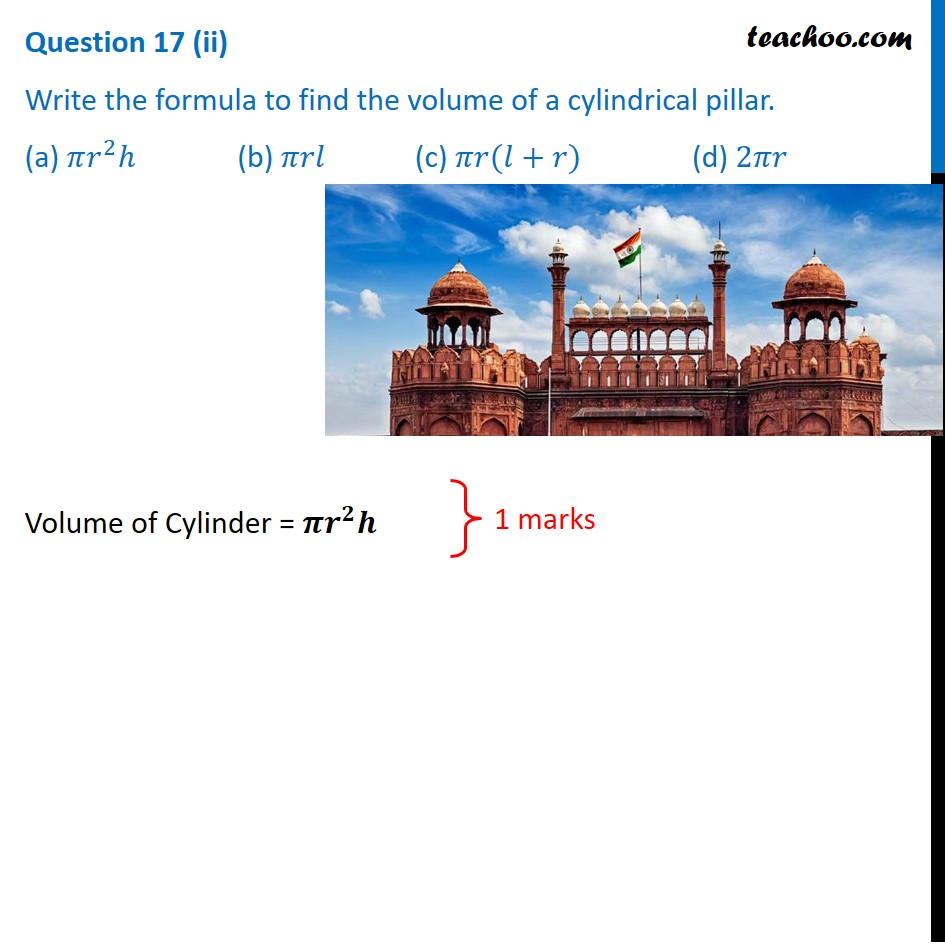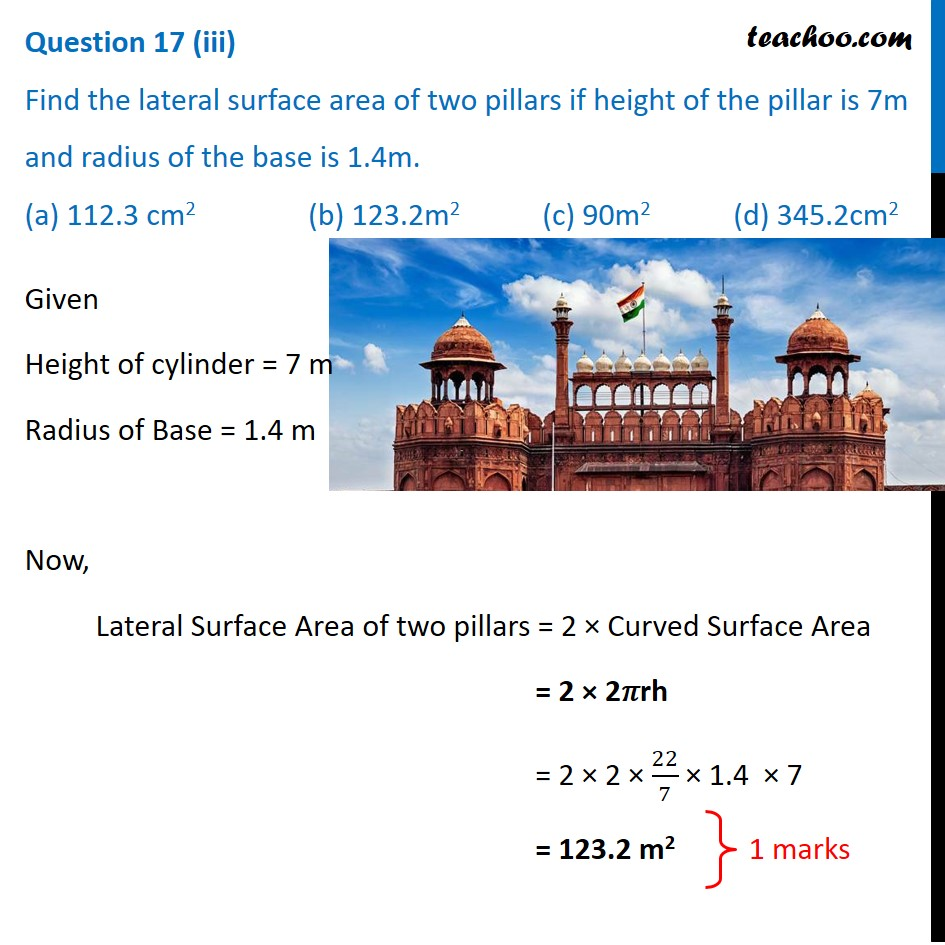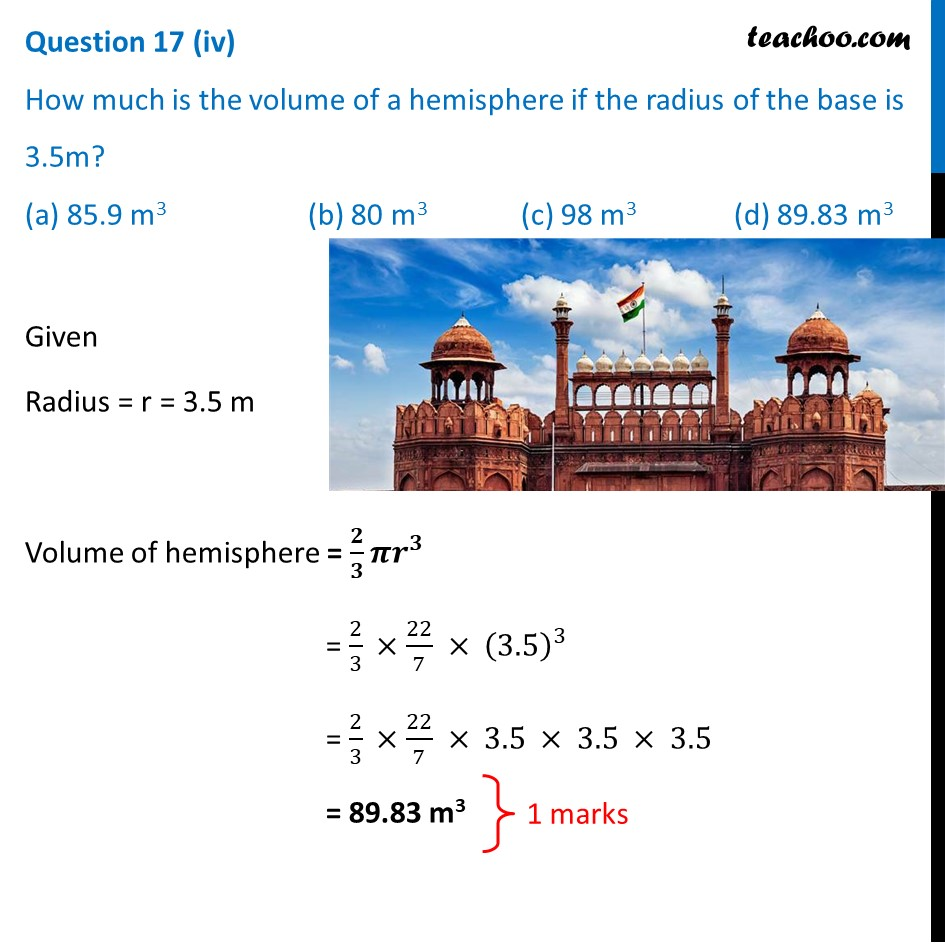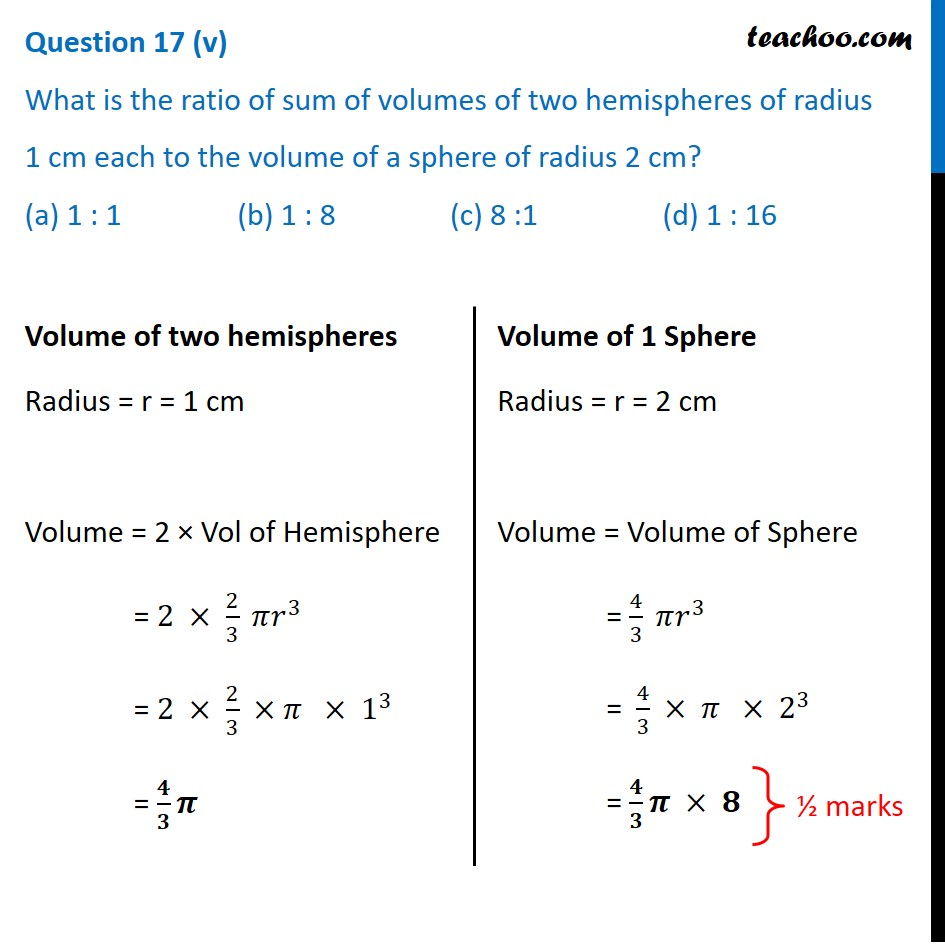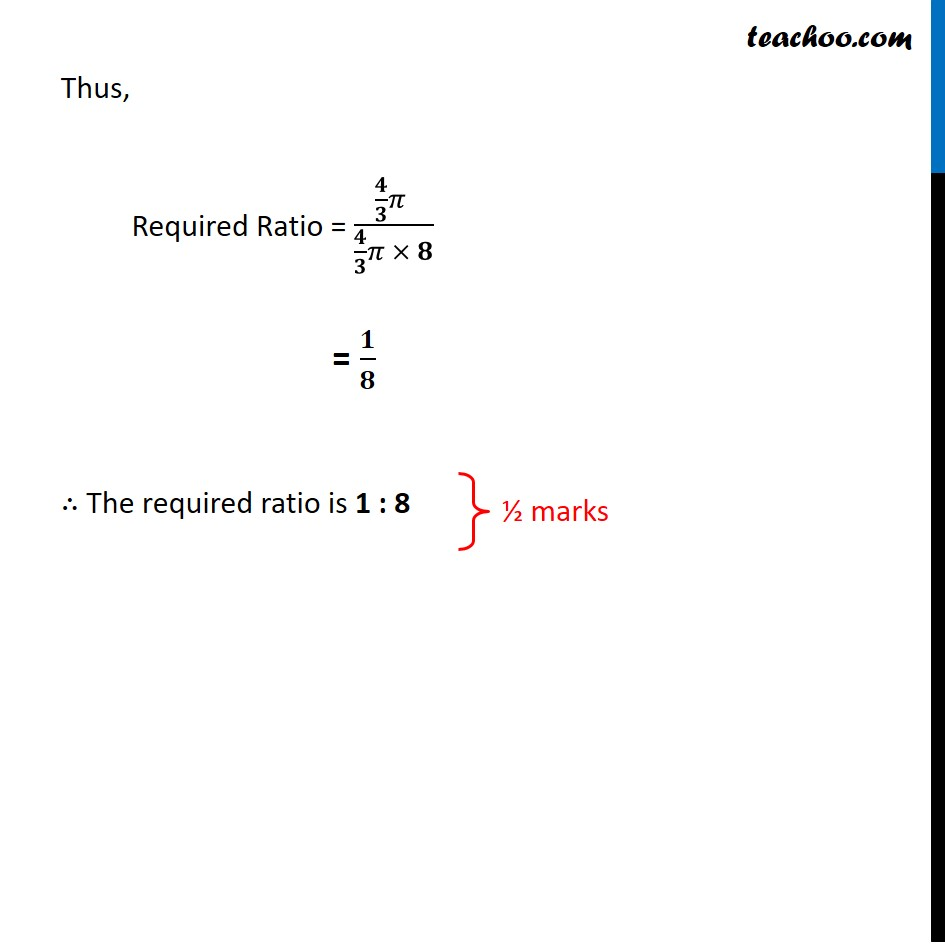1. Class 10
2. Solutions of Sample Papers for Class 10 Boards
3. CBSE Class 10 Sample Paper for 2021 Boards - Maths Basic

Transcript

Question 17 Mathematics teacher of a school took her 10th standard students to show Red fort. It was a part of their Educational trip. The teacher had interest in history as well. She narrated the facts of Red fort to students. Then the teacher said in this monument one can find combination of solid figures. There are 2 pillars which are cylindrical in shape. Also 2 domes at the corners which are hemispherical.7 smaller domes at the centre. Flag hoisting ceremony on Independence Day takes place near these domes. Question 17 (i) How much cloth material will be required to cover 2 big domes each of radius 2.5 metres? (Take 𝜋 = 22/7) (a) 75 m2 (b) 78.57m2 (c) 87.47m2 (d) 25.8m2 Domes are Hemisphere of radius 2.5 cm ∴ r = 2.5 cm Cloth material to cover would be Surface Area of Hemisphere of both domes ∴ Cloth material = 2 × Surface Area of Hemisphere = 2 × 2𝜋r2 = 2 × 2 × 22/7 × (2.5)2 = 78.57 m2 Question 17 (ii) Write the formula to find the volume of a cylindrical pillar. (a) 𝜋𝑟^2 ℎ (b) 𝜋𝑟𝑙 (c) 𝜋𝑟(𝑙+𝑟) (d) 2𝜋𝑟. Volume of Cylinder = 𝝅𝒓^𝟐 𝒉 Question 17 (iii) Find the lateral surface area of two pillars if height of the pillar is 7m and radius of the base is 1.4m. (a) 112.3 cm2 (b) 123.2m2 (c) 90m2 (d) 345.2cm2 Given Height of cylinder = 7 m Radius of Base = 1.4 m Now, Lateral Surface Area of two pillars = 2 × Curved Surface Area = 2 × 2𝜋rh = 2 × 2 × 22/7 × 1.4 × 7 = 123.2 m2 Question 17 (iv) How much is the volume of a hemisphere if the radius of the base is 3.5m? (a) 85.9 m3 (b) 80 m3 (c) 98 m3 (d) 89.83 m3 Given Radius = r = 3.5 m Volume of hemisphere = 𝟐/𝟑 𝝅𝒓^𝟑 = 2/3 ×22/7 × (3.5)^3 = 2/3 ×22/7 × 3.5 × 3.5 × 3.5 = 89.83 m3 Question 17 (v) What is the ratio of sum of volumes of two hemispheres of radius 1 cm each to the volume of a sphere of radius 2 cm? (a) 1 : 1 (b) 1 : 8 (c) 8 :1 (d) 1 : 16 Volume of two hemispheres Radius = r = 1 cm Volume = 2 × Vol of Hemisphere = 2 × 2/3 𝜋𝑟^3 = 2 × 2/3 ×𝜋 × 1^3 = 𝟒/𝟑 𝝅 Volume of 1 Sphere Radius = r = 2 cm Volume = Volume of Sphere = 4/3 𝜋𝑟^3 = 4/3 × 𝜋 × 2^3 = 𝟒/𝟑 𝝅 × 𝟖 Thus, Required Ratio = (𝟒/𝟑 𝜋 )/(𝟒/𝟑 𝜋 × 𝟖) = 𝟏/𝟖 ∴ The required ratio is 1 : 8

CBSE Class 10 Sample Paper for 2021 Boards - Maths Basic

Class 10
Solutions of Sample Papers for Class 10 Boards